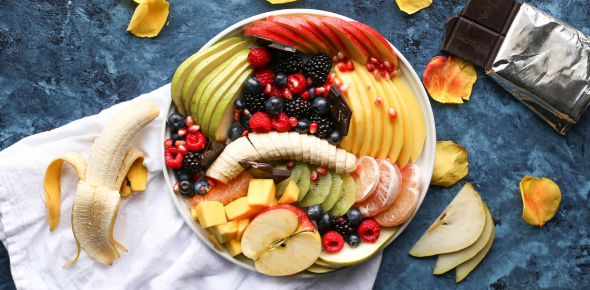# Nutrition Facts Labels: Trivia Quiz!

10 Questions | Total Attempts: 681Settings.

• 1.
This is a sample label from a can of corn kernels What is the total content of corn in this can, in grams?
• A.

85g

• B.

255g

• C.

306g

• D.

100g

• 2.
This is a sample label from a can of corn kernels If you consumed the whole can of corn, what would be your total intake of saturated fat, in grams?
• A.

0.5g

• B.

0.3g

• C.

0.9g

• D.

1.1g

• E.

0.09g

• 3.
This is a sample label from a can of corn kernels How much protein is there in 100g of corn?
• A.

2.2g

• B.

6.6g

• C.

7.8g

• D.

2.6g

• 4.
This is a sample label from a can of corn kernels If you consumed the entire can of corn, would this be considered high, moderate or low in terms of energy intake?
• A.

Moderate

• B.

High

• C.

Low

• 5.
This is a sample label from a can of corn kernels Would the amount of saturated fat in this can of corn be considered acceptable for a healthy diet?
• A.

Yes

• B.

No

• 6.
This is a sample label from a can of corn kernels Approximately how many teaspoons of sugar are there in one serving of this corn?
• A.

5 teaspoons

• B.

4 teaspoons

• C.

1 teaspoon

• 7.
This is a sample label from a can of corn kernels Would this can of corn be considered a good source of fiber?
• A.

No

• B.

Yes

• 8.
This is a sample label from a can of corn kernels If you consumed the whole can of corn, approximately what percentage of your daily intake of sodium would you consume?
• A.

30%

• B.

10%

• C.

100%

• D.

115%

• 9.
This is a sample label from a can of corn kernels Would the %DV (daily value) of carbohydrate in one serving of this can of corn be considered high or low?
• A.

High

• B.

Low

• 10.
If a label claims that the food is 98% fat-free, and it has 500 Cals, would this be a true fat-free food?
• A.

Yes

• B.

No

Related TopicsBack to top